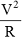# Physics

### Chapter : 1. Electricity

#### Electric Energy

Electric Energy When a potential difference is applied across a wire, current starts flowing in it. The free electrons collide with the positive ions of the metal and lose energy. Thus energy taken from the battery is dissipated. The battery constantly provide energy to continue the motion of electron and hence electric current in the circuit. This energy is given to ions of the metal during collision and thus temperature of wire rises. Thus, energy taken from the battery gets transferred in to heat. This energy is called electrical energy. This effect is also called 'Heating Effect of Current'.
If > R = Resistance of wire
I = Current in wire
V = Potential difference across wire.
Flow of charge in 't' time = It.
Energy dissipated W = Vq = VIt,
&beacuse; V = IR
∴ W = VIt = I2Rt =t = Vq in Jule
This energy is equal to work done by battery or heat produced in the wire.